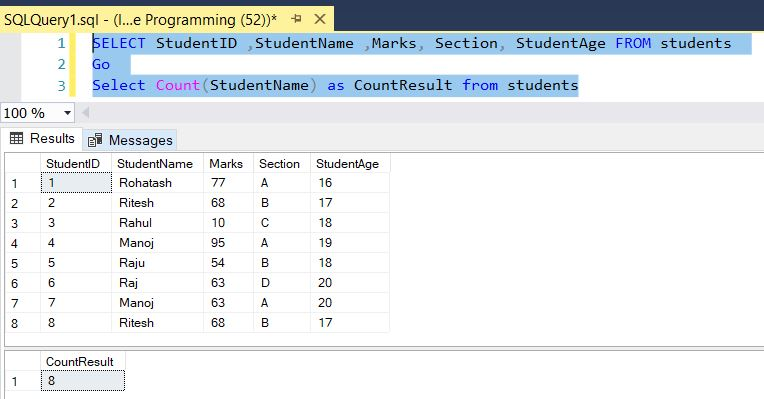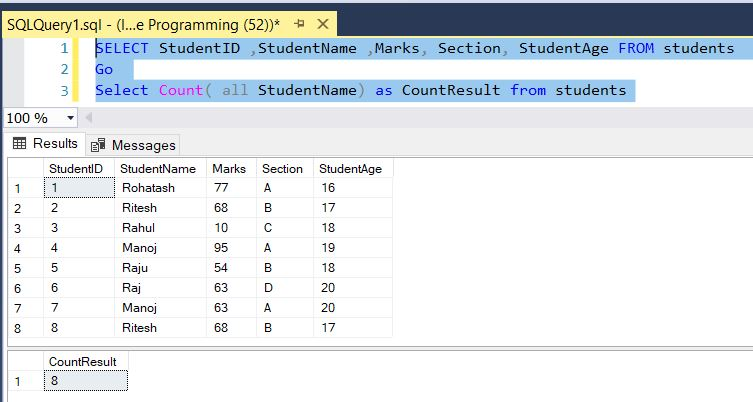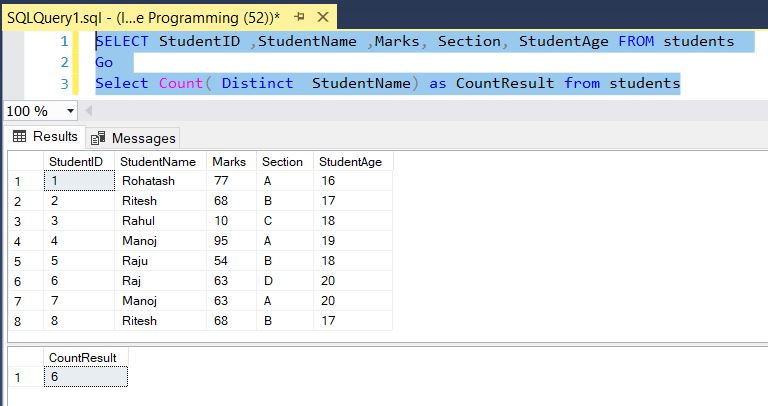﻿ SQL Count vs CountALL - TutorialsTrend
This Angular tutorial helps you get started with Angular quickly and effectively through many practical examples.
`

# SQL - Count vs CountALL

In this article, you consider the Count function which is used to count the number of rows in a database table and will see the diffrence between count and count all function with the help of example.

#### Count vs Count All Function

Count(All) and Count(Distinct) are two aggregate functions in SQL Server. Count function is a part of the SQL Server's aggregate functions. In aggregates, we consider various types of functions like count, max, avg, min, and sum. In this article, you consider the Count function which is used to count the number of rows in a database table.

Let's have a look at a practical example of how to get the difference between the Count(All) and Count(Distinct) in SQL Server. The example is developed in SQL Server 2012 using the SQL Server Management Studio.

#### Count() Function in SQL Server

The COUNT() function is used to count the number of rows when you use a where condition with the Count function in a select statement. It will return the number of rows from the table satisfying the where condition. NULL values will not be counted by the Count function.

Example

``````SELECT StudentID ,StudentName ,Marks, Section, StudentAge FROM students
Go
Select Count(StudentName) as CountResult from students``````

Output#### Count(All ColumnName) Function

The COUNT(All ColumnName) function is similar to Count(ColumnName). It also produces the same result as Count(ColumnName).

Example

``````SELECT StudentID ,StudentName ,Marks, Section, StudentAge FROM students
Go
Select Count(all StudentName) as CountResult from students``````

Output#### Count(Distinct ColumnName) Function

When the DISTINCT keyword is used, all duplicate values are eliminated before the function count is applied, or if you want to count the number of unique rows in the table. This form of Count does not count rows with null values for the column.

Example

``````SELECT StudentID ,StudentName ,Marks, Section, StudentAge FROM students
Go
Select Count(Distinct StudentName) as CountResult from students``````

OutputNext